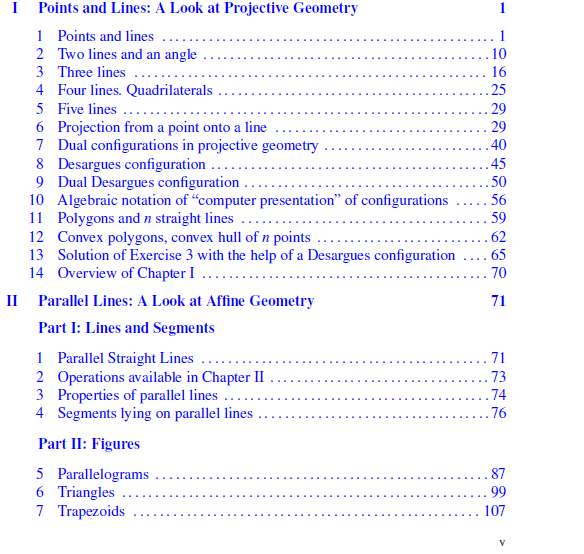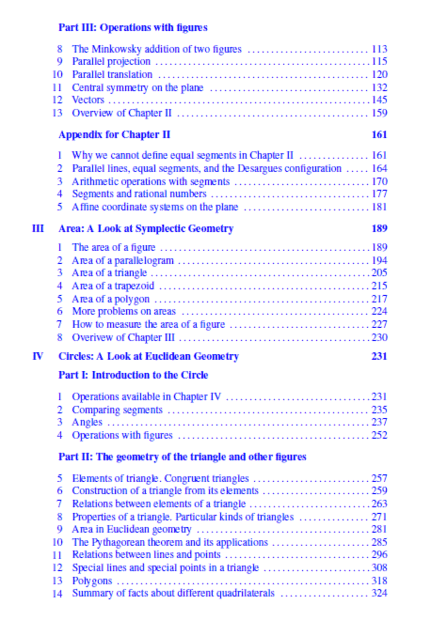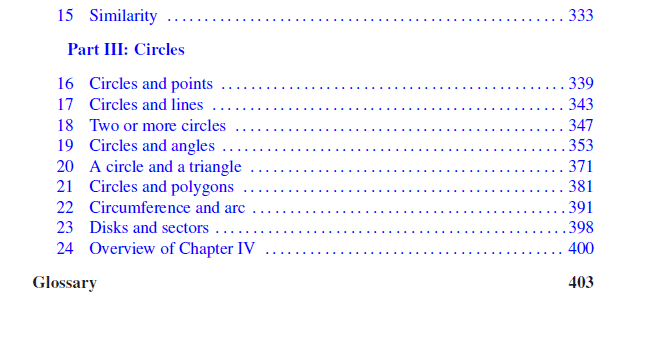# Surface that when superimposed takes any one point to any other point

• B
simphys
TL;DR Summary
Give an example of a surface other than the plane which, like
the plane, can be superimposed on itself in a way that takes any one
given point to any other given point.
I don't get what is meant with the last part: that takes any one
given point to any other given point.

Homework Helper
Gold Member
2022 Award
I don't get what is meant with the last part: that takes any one
given point to any other given point.
It's not very precise. But, I can guess what they mean.

Hint: think of maximal symmetry.

•simphys
simphys
It's not very precise. But, I can guess what they mean.

Hint: think of maximal symmetry.
aaah so for example a sphere which is symmetric in any way possible?

Thanks!

simphys
@PeroK a quick one in between, if it stated to show that f.e. for any two points of a plane, there is a line in this plane passing through the point and that such line is unique. Does this mean to construct a drawing to show that this case/definition is true or?

Homework Helper
Gold Member
2022 Award
@PeroK a quick one in between, if it stated to show that f.e. for any two points of a plane, there is a line in this plane passing through the point and that such line is unique. Does this mean to construct a drawing to show that this case/definition is true or?
I suspect they want you to prove it! Perhaps using the geometric properties of the plane to simplify the proof.

simphys
I suspect they want you to prove it! Perhaps using the geometric properties of the plane to simplify the proof.
ah, well i guess I am screwed at this point then lol 😬

Homework Helper
Gold Member
2022 Award
ah, well i guess I am screwed at this point then lol 😬
Can you see how it might be done for the x-y plane? Try it for a specific pair of points first. Such as the points ##(1, 2)## and ##(5, 3)##. Is there a straight line between those points? Is it unique?

simphys
Can you see how it might be done for the x-y plane? Try it for a specific pair of points first. Such as the points ##(1, 2)## and ##(5, 3)##. Is there a straight line between those points? Is it unique?
by the axiom that a line can be formed through two points which is said to be unique. we know that the line is unique.
And then we also know that as those two points lie in the plane, the straight line will also line in the plane going through those two points.

simphys
Is this enough to state this together with a drawing that shows that the line through two points lies in the plane?

Homework Helper
Gold Member
2022 Award
by the axiom that a line can be formed through two points which is said to be unique. we know that the line is unique.
And then we also know that as those two points lie in the plane, the straight line will also line in the plane going through those two points.
It's all very well to quote a Euclidean axiom in this case, but if you have to be prepared at some point to move on to algebraic and vector-based geometry.

simphys
It's all very well to quote a Euclidean axiom in this case, but if you have to be prepared at some point to move on to algebraic and vector-based geometry.
Theen I can say that m = (3-2) / (5-1) with y - 5 = m(x-3) => y = x/4 + 17/4 which will form a straight line through those two points, like this?

simphys
It's all very well to quote a Euclidean axiom in this case, but if you have to be prepared at some point to move on to algebraic and vector-based geometry.
I get what you mean, but I went through analytic geometry and use vector-based geometry (in physics I suppose then) but haven't learned this elementry geometry which the others build on though

Homework Helper
Gold Member
2022 Award
Theen I can say that m = (3-2) / (5-1) with y - 5 = m(x-3) => y = x/4 + 17/4 which will form a straight line through those two points, like this?
Exactly. Algebraically, the lines in the plane are defined by ##y = mx + c## and ##x = a##.

Homework Helper
Gold Member
2022 Award
I get what you mean, but I went through analytic geometry and use vector-based geometry (in physics I suppose then) but haven't learned this elementry geometry which the others build on though
I never studied pure Euclidean axiomatic geometry. It's a bit of a specialist area these days.

•simphys
simphys
I never studied pure Euclidean axiomatic geometry. It's a bit of a specialist area these days.
for real?! I haven't myself as well! But in physics, I feel that I miss all these topics that come up in calc/intro physics (Newtonian mechanics,...)

okay but let me clarify have you studied basic geometry, like this you know about lines, geometric solid, figures, concurrency, triagnles simliarities econgruencies,... or not?

simphys
@PeroK, I asked here, advice was to go through geometry, algebra etc as it's fundamental. But if not pure geometry what book should I use instead this to improve at it

Homework Helper
Gold Member
2022 Award
@PeroK, I asked here, advice was to go through geometry, algebra etc as it's fundamental. But if not pure geometry what book should I use instead this to improve at it
I don't have a recommendation for a geometry textbook. The Kiselev looks inappropriate to me. The Selby book looks better, although try not to get bogged down in it.

simphys
I don't have a recommendation for a geometry textbook. The Kiselev looks inappropriate to me. The Selby book looks better, although try not to get bogged down in it.
Arghh, there goes a wasted day haha -.-
anyways, thanks a lot!
And yep will try to, I'll go through it in parallel with physics and calc and see what happens.

Homework Helper
Gold Member
2022 Award
okay but let me clarify have you studied basic geometry, like this you know about lines, geometric solid, figures, concurrency, triagnles simliarities econgruencies,... or not?
Yes, obviously. But, you don't want to get bogged down in geometry as a formal pure mathematical discipline - unless you want to. I'd draw an analogy with number theory. That's a specific discipline.

For example, you may take as a postulate that the shortest distance between two points is a straight line, but eventually you'll use the calculus of variations to prove it.

PS The Gelfand looks like the sort of thing you want. But, Selby is a free pdf, so why not take a look at that first?

•simphys
simphyssimphys
Yes, obviously. But, you don't want to get bogged down in geometry as a formal pure mathematical discipline - unless you want to. I'd draw an analogy with number theory. That's a specific discipline.

For example, you may take as a postulate that the shortest distance between two points is a straight line, but eventually you'll use the calculus of variations to prove it.

PS The Gelfand looks like the sort of thing you want. But, Selby is a free pdf, so why not take a look at that first?
both freee :) gelfand's one

simphys
Yes, obviously. But, you don't want to get bogged down in geometry as a formal pure mathematical discipline - unless you want to. I'd draw an analogy with number theory. That's a specific discipline.

For example, you may take as a postulate that the shortest distance between two points is a straight line, but eventually you'll use the calculus of variations to prove it.

PS The Gelfand looks like the sort of thing you want. But, Selby is a free pdf, so why not take a look at that first?
Aah like that, I get it, okay thanks a lot! so basically for 'our' purpose (referring to physical) it is kind sort of useless in a sense as calculus is more of the basis of physics and gives easier methods of approaching things
Thanks a lot for the advice, I really appriace it a whole lot!
(by the way.. I refer to that websites but most of the time just to look at the books so that I can buy them)

Homework Helper
Gold Member
2022 Award
•simphys
simphys
Looks good to me.
Thanks a lot for the help @PeroK , I really appreciate it! I almost went onto the disarter path lol...
Oh one more thing, if you say try not to get bogged down with the ''pureness'' does this same thing count for calculus' proofs aswell? (besides perhaps those of continuity,... and fundamental theoreom of calculus)

•PeroK
Homework Helper
Gold Member
2022 Award
Oh one more thing, if you say try not to get bogged down with the ''pureness'' does this same thing count for calculus' proofs aswell? (besides perhaps those of continuity,... and fundamental theoreom of calculus)
It's a good question. You need to know something about the theory of limits and calculus. But, it's a lot of effort to master Real Analysis. There's a website I like for all things calculus and I think he strikes a good balance between the applied mathematics and the necessary theoretical foundations:

https://tutorial.math.lamar.edu/

•simphys
The calculus reasoning may go sort of like this: Let $\Phi$ be a bijective (one-to-one and onto) mapping from the plane to a surface. Let a and b be the two points we want mapped. Let $\Psi$ be the mapping that takes $\Phi^{-1}(a)$ to $\Phi^{-1}(b)$ . Then $\Phi\circ\Psi\circ\Phi^{-1}$ maps a to b.

•simphys
simphys
It's a good question. You need to know something about the theory of limits and calculus. But, it's a lot of effort to master Real Analysis. There's a website I like for all things calculus and I think he strikes a good balance between the applied mathematics and the necessary theoretical foundations:

https://tutorial.math.lamar.edu/
Oh yeah I've seen these before, Thanks I will definitely use them, even for multivariable! And thanks for the answer once again.

To give you a perspective of why I asked this question:
This is what I did was.. as calc1 from university was also kinda skipping a couple of important things (f.e. exterma). I bought thomas Calculus 13 th edition and used that in parallel but kept getting bogged down too much into those unnecessary proofs like those from exponentials and logs etcetera and there we go again.. I am not really getting the conceptual idea as I wasn't in physics :'D besides now having the ability to do the computational side ofc.

So at this point I bought calculus with applications from peter lax now, will give me a quick revise and is more application oriented with separate application sections.
This time however, I'll just structure it with notes and review those notes, seems more efficient than what I was doing till now.

simphys
The calculus reasoning may go sort of like this: Let $\Phi$ be a bijective (one-to-one and onto) mapping from the plane to a surface. Let a and b be the two points we want mapped. Let $\Psi$ be the mapping that takes $\Phi^{-1}(a)$ to $\Phi^{-1}(b)$ . Then $\Phi\circ\Psi\circ\Phi^{-1}$ maps a to b.
Yeah that is basically the 'definitions' of the theoremes than basically, right? I without a doubt was focusing waaay too much on the proves than needed, especially for first-time learning